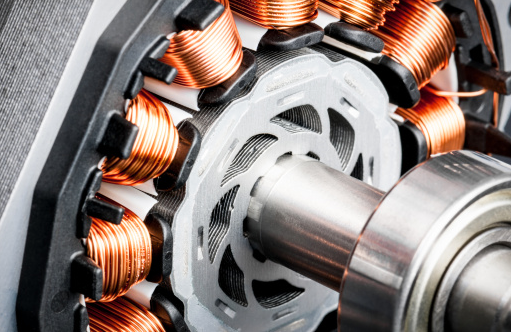## Motor Poles

An induction motor operates with a 0.20 slip, and a line voltage frequency of 120 Hz. If the motor shaft's rotational speed is 11,520 rpm, how many pole(s) does it have?Hint
The synchronous speed for ac motors is:
$$n_s=120*f/p$$$where $$f$$ is the line voltage frequency in Hz, and $$p$$ is the number of poles. Hint 2 The slip for an induction motor is: $$slip=\frac{(n_s-n)}{n_s}$$$
where $$n$$ is the rotational speed, and $$n_s$$ is the synchronous speed.
The slip for an induction motor is:
$$slip=\frac{(n_s-n)}{n_s}$$$where $$n$$ is the rotational speed, and $$n_s$$ is the synchronous speed. Solving for synchronous speed: $$(n_s)slip=n_s-n$$$
$$n=n_s-(n_s)slip$$$$$n=n_s(1-slip)$$$
$$n_s=\frac{n}{(1-slip)}=\frac{11,520rpm}{1-0.20}=\frac{11,520rpm}{0.80}=14,400\:rpm$$$The synchronous speed for ac motors is: $$n_s=120*f/p$$$
where $$f$$ is the line voltage frequency in Hz, and $$p$$ is the number of poles.
Solving for number of poles:
$$p=\frac{120\cdot f}{n_s}=\frac{120(120)}{14,400rpm}=\frac{14,400}{14,400}=1$$\$
1